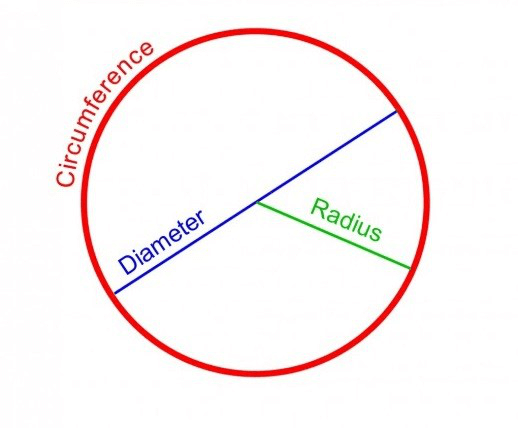# Circle Area Calculator

Enter Information

#### Result

Fill the calculator form and click on Calculate button to get result here

## Area of a Circle Calculator

The area of a circle calculator is an online tool that is used to calculate multiple values related to a circle.
The circle calculator can find followings with steps:

• Area of a circle
• Diameter of circle
Circumference of a circle

After calculating the area of a circle, you can also calculate the sector of a circle using our sector area calculator.

## What is a Circle?

Khan Academy defines a circle as:

A circle is a shape where the distance from the center to the edge of the circle is always the same. Every circle has a center, which is a point that lies at the center of the circle.Radius: The distance from the center of the circle to its boundary is called the radius of the circle. It is denoted by r.

Diameter: A distance or line from the center of the circle that touches the boundary of the circle on two sides is called the diameter of the circle. It is denoted by d. The diameter is exactly double the radius.

Circumference: The distance around the circle is called the circumference of the circle. It is denoted by C.

## Area of a Circle Formula

The formula to find the area of a circle is very simple to interpret and use. If we take the square of the radius of the circle and multiply it with pi ($$pi$$), we will get the circle area.

The area of a circle formula can be expressed as:

A = --\pi r^2--

Where:

A represents the area of the circle,

r is the radius of the circle,

and π is the mathematical constant valuing 13.1415.

## How to Find the Area of a Circle?

The surface area of a circle calculator above can be used to find the area of a circle with much comfort.
However, if you need to find the circle area manually without using any circle area calculator, you can follow the below steps:

• Determine the radius of the circle and write it down.
• Write down the area of the circle formula.
• Substitute the values in the formula and calculate the area.
Let’s go try this method by using an example.

### Example:

If the radius of a circle is 20 cm, find the area of that circle.

Solution:

Step 1: Determine the radius of the circle and write it down.

r = $$20$$

Step 2:

Write down the area of the circle formula.

A = $$\pi r^2$$

Step 3:

Substitute the values in the formula and calculate the area.

A = $$3.1415\times 202$$

A = 1256.6 cm2

So, the area of the circle with a 20 cm radius will be 1256.6 cm2.

A manual of greek mathematics | source by HEATH THOMAS L (2003)
Area of a circle explained | source by Wikipedia.com
Circumference and area of a circle - Perimeter and area - National 4 Application of Maths | BBC.CO.UK

Other Languages
User Ratings
• Total Reviews 0
• Overall Rating 0/5
• Stars
Reviews
No Review Yet